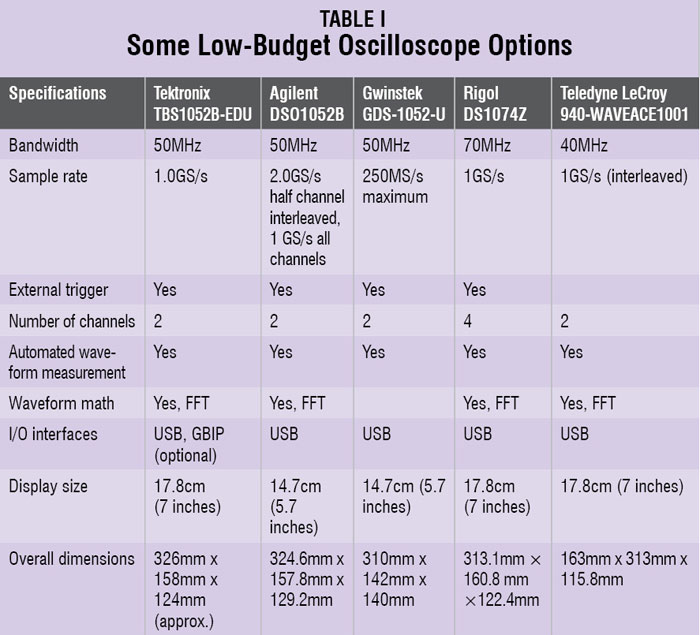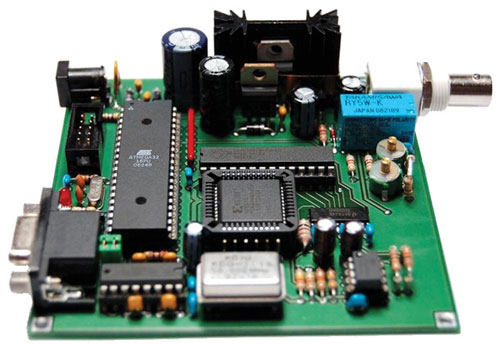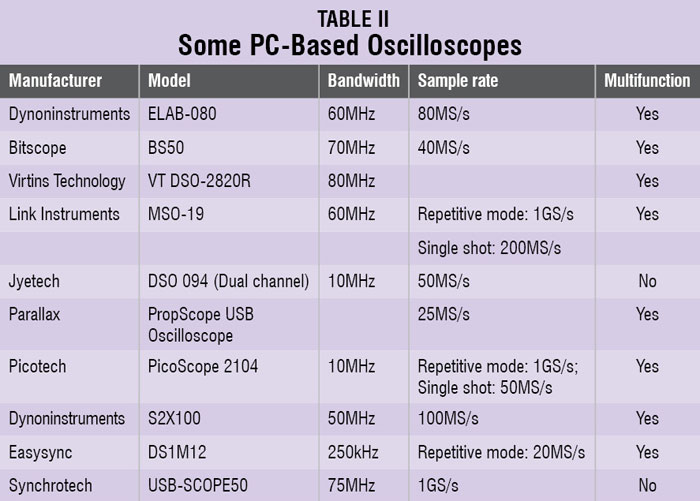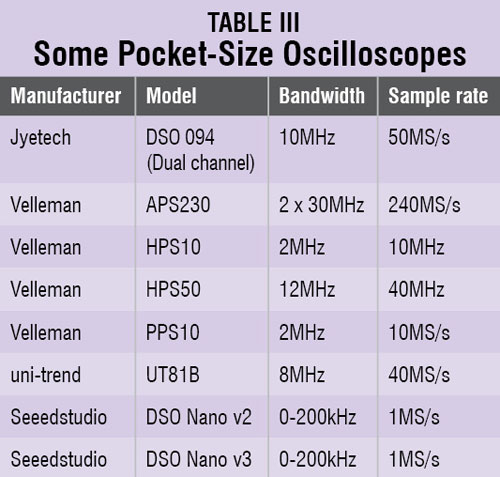There are also various open source do it yourself (DIY) oscilloscope designs available on the Internet. You can build these systems yourself and use for basic test and measurement. Such systems are very useful for students as they learn a lot while building their own oscilloscope and then use it with better confidence. Fig. 4 shows such a system.Fig. 3: A pocket-size oscilloscopeFig. 4: A DIY-type oscilloscope

There are so many options available for each type of oscilloscope. But before looking at these options to evaluate which one suits your requirement, you need to understand their specifications. You can have correct measurements only when your oscilloscope’s specifications support the range of the signals you are trying to measure. Some of the important specifications are:

Bandwidth. It determines the maximum frequency signal that the oscilloscope can accurately measure. The accuracy decreases with increase in signals’ frequency. The bandwidth mentioned in the datasheet (say, 100MHz) is actually the frequency at which a sinusoidal input is attenuated to 70.7% of its true amplitude. Beyond this frequency the oscilloscope cannot support reasonable accuracy. To decide how much bandwidth you need, find out what would be the range of frequencies you would need to measure. Once you know the frequency range, just use the ‘five times’ rule, that is, multiply the maximum frequency by five and you have the bandwidth that you need to accurately measure those signals.Rise time. Rise time is the time taken by a signal to change from a specified low value to a specified high value. Typically, these values are 10% and 90% of the step height. As a thumb rule, similar to bandwidth, the rise time of the oscilloscope should be less that 1/5 of the fastest rise time of the signal to accurately measure it.

Sample rate. Sample rate refers to how frequently a digital oscilloscope takes a sample of the signal. The faster an oscilloscope samples, lesser the details lost while reconstructing the signal. In order to accurately reconstruct a signal and avoid aliasing, the Nyquist theorem states that the signal must be sampled at least twice as fast as its highest frequency component. This theorem assumes an infinite record length and a continuous signal, but no oscilloscope can offer infinite record length. Therefore sampling at only twice the rate of the highest frequency component will not be sufficient.

The sampling rate that you might require to accurately read your signal will majorly depend on the method used for reconstructing the signal, also called interpolation. For accurate reconstruction using sin(x)/x interpolation, sample rate should be at least 2.5 times the highest frequency component of your signal. Using linear interpolation, the sample rate should be at least 10 times the highest frequency signal component.

Record length. The oscilloscope cannot store infinite number of samples as assumed by Nyquist equation. Record length determines the time that can be captured by each channel of the oscilloscope.

Time captured = Record length/Sample rate

Normally, an entry-level oscilloscope comes with 2k to 2.5k points, which is more than enough. In general, greater record length is better. To capture and debug serial bus you will need record length as high as 1M points.